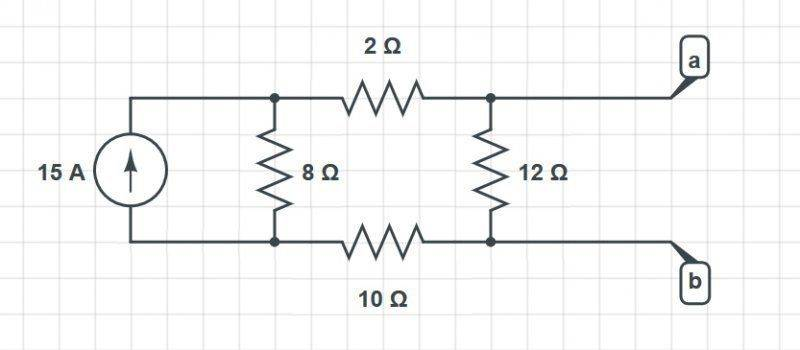# Voltage across terminals

## Homework Statement

Task: Find the voltage across the terminals a and b in the circuit (Done).
My question: Why does the solution work?

## Homework Equations

Current division
ix = Isource * Gbranch/Gequivalent

## The Attempt at a Solution

The voltage across (or is it between ?) the terminals is the same (why ?) as the voltage drop at the branch with the 2, 12 and 10 Ohm resistors, which is 45 Volts. This was found by first finding the current flowing through the branch with the current division principle, i = 3.75 A.

As I stated above, why is the voltage across the terminals the same as the voltage drop at the branch with the 2, 12 and 10 Ohm resisotrs?

The circuit is attached below.gneill
Mentor

## Homework Statement

Task: Find the voltage across the terminals a and b in the circuit (Done).
My question: Why does the solution work?

## Homework Equations

Current division
ix = Isource * Gbranch/Gequivalent

## The Attempt at a Solution

The voltage across (or is it between ?) the terminals is the same (why ?) as the voltage drop at the branch with the 2, 12 and 10 Ohm resistors, which is 45 Volts. This was found by first finding the current flowing through the branch with the current division principle, i = 3.75 A.

As I stated above, why is the voltage across the terminals the same as the voltage drop at the branch with the 2, 12 and 10 Ohm resisotrs?

The circuit is attached below.

View attachment 78771
Hi Gecata, Welcome to Physics Forums.

Yes, voltage (or potential difference) is measured between two points or across a component. Current goes though a wire or component.

Perhaps there's a terminology issue, but I 'm not entirely clear on your problem. Can you show your work?

Actually I see the answer to my question now (not really a question apparently).
The terminals A and B are open, and I needed to determine the resistance between them, in order to model a Thevenin and Norton equivalent circuits.

The confusing momenta was not really paying attention to terminals A and B being open.

CWatters